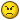## Recommended Posts

If you had a mole of carbon atoms and weighed it on a scale, what would you see? I guess what I should ask is what does a scale measure, weight or mass? If you measured that mole of carbon atoms, would the scale read 12 or would it read 12 x 9.8 ? If it reads 12, does that mean that the scale automatically divides out the 9.8 to get the mass?

##### Share on other sites

a mole of carbon atoms weighs 12 grams.

theres nothing complicated involved##### Share on other sites

1 mole of carbon atoms will have a mass of just over 12 - i.e its atomic mass.

The general conversion formula for this is:

Moles = Mass (g) / rmm/ram

where are you getting the 9.8 from?

##### Share on other sites

If you had a mole of carbon atoms and weighed it on a scale, what would you see? I guess what I should ask is what does a scale measure, weight or mass? If you measured that mole of carbon atoms, would the scale read 12 or would it read 12 x 9.8 ? If it reads 12, does that mean that the scale automatically divides out the 9.8 to get the mass?

A mole of C-12 atoms will have a mass of 12 grams (you'll have some C-13 and possibly C-14 in a natural sample of carbon)

What the scale reads depends on the scale. A balance scale will give you 12 grams (modified by the tiny bouyancy force which you probably won't see). A spring scale will measure the normal force, which is also modified by the rotation of the earth (a centrifugal force if you're in the rotating frame). The actual readout depends on how it was made and calibrated. It could read out in kg, N, lbs, slugs, stone, etc.

##### Share on other sites

Ah, ok. Thanks for clearing that up. So the scales I'm thinking of in the lab are made to read out in mass. I was thinking that scales were made to read out the weight of an object (like bathroom scales).

The 9.8 is for 9.8 m/s^2 from acceleration of gravity. I was thinking that the number on the scale meant the weight and not the mass.

"a mole of carbon atoms weighs 12 grams.

theres nothing complicated involved :)"

I think the confusing thing is that the word "weigh" is used as a verb for "to find the mass of" and "to find the weight of".

##### Share on other sites

m*g = F, not weight. Stop dragging physics in simple chemistry equations##### Share on other sites

I think the confusing thing is that the word "weigh" is used as a verb for "to find the mass of" and "to find the weight of".

Yes, they are sometimes interchanged and shouldn't be. (kilo)grams and slugs are mass, newtons and lbs are weight. Engineers will use lbs-mass which still makes me cringe; I think it leads to sloppy thinking. I was never comfortable with the "plug and chug" approach to formulae I saw in the few courses I took from engineering professors.

##### Share on other sites

I agree there is indeed quite a distinction between mass and weight, but for Chem purposes, Weight is the Preferred term.

maybe STP should be upgraded to STP&G (Standard room Temperature and Pressure & Gravity).

Just Kidding## Create an account

Register a new account